• 一种基于SDN的BBU-RRH动态分配算法，龙恳，赵勇，在云无线接入网（C-RAN）中，一个基带单元（BBU）可以为一组有限的射频拉远头（RRH）提供服务，BBU和RRH之间的逻辑连接应该是动态的，�
• 操作系统课设做的动态分区分配算法。第一次上传资源，做的有些乱，献丑了，其中循环首次循环和最佳、最坏分配算法其实只是从首次适应算法改了一点东西。 补充几句，是JAVA做的，分配和回收算法都有，使用数组实现
• 动 态 内 存 分 配 算 法 实 验 报 告 院系:计算机与通信工程学院 班级:计科08-1班 姓名:胡太祥 学号:200807010112 一实验题目动态内存分配算法 二实验目的 深入了解动态分区存储管理方式内存分配与回收的实现 三...
• 1，算法思想：由于动态分区分配是一种连续分配方式，为各个进程分配的空间必须是一整片区域，因此为了保证“大进程”到来时能有连续的大片空间，可以尽可能地留下大片空闲区，优先使用更小的空闲区。 2，如何实现...

一，首次适应算法（First Fit）

1，算法思想：每次都从低地址部分开始查找，找到第一个能满足大小的空闲分区

2，如何实现：空闲分区一地址递增的次序排列，每次分配内存时顺序查找空闲分区表，找到大小能满足要求的第一个空闲分区

二，最佳适应算法（Best Fit）

1，算法思想：由于动态分区分配是一种连续分配方式，为各个进程分配的空间必须是一整片区域，因此为了保证“大进程”到来时能有连续的大片空间，可以尽可能地留下大片空闲区，优先使用更小的空闲区。

2，如何实现：空闲区按容量递增次序连接，每次分配内存时顺序查找空闲分区链，找到大小能满足要求的第一空闲分区。

3，缺点：每次都选最小的分区进行分配，会留下越来越多，很小的，难以利用的内存块。因此这种方法会产生很多外部碎片

三，最坏适应算法（Worst Fit）

1，算法思想：为了解决最佳适应算法的问题——留下太多难以利用的小碎片，可以在每次分配时优先使用最大的连续空闲区，这样分配后剩余的空闲区就不会太小，更方便使用

2，如何实现：空闲区按容量递减的次序连接，每次分配内存时顺序查找空闲区，找到第一个可以满足要求的空闲分区。

3，缺点：每次都选最大的分区进行分配，虽然可以让分配后留下的空闲区更大，更可用，但是这种方式会导致较大的连续空闲区被迅速用完。如果之后有“大进程”到达，就没有内存可用了

四，邻近适应算法（Next Fit）

1，算法思想：首次适应算法每次都从链头开始查找，这可能会导致低地址部分出现很多很小的空闲分区，二每次分配查找时，都要经过这些分区，因此也增加了查找的开销。如果每次都从上次查找结束的位置开始检索，就能解决上述问题。

2，如何实现：空闲分区已地址递增的顺序排列（可以排列成一个循环链表）每次分配内存时从上次查找结束的位置开始查找空闲分区链，找到大小能满足要求的第一个空闲分区

3，缺点：可能会导致高地址部分的大分区更可能被使用，划分为小分区，最后导致无大分区可用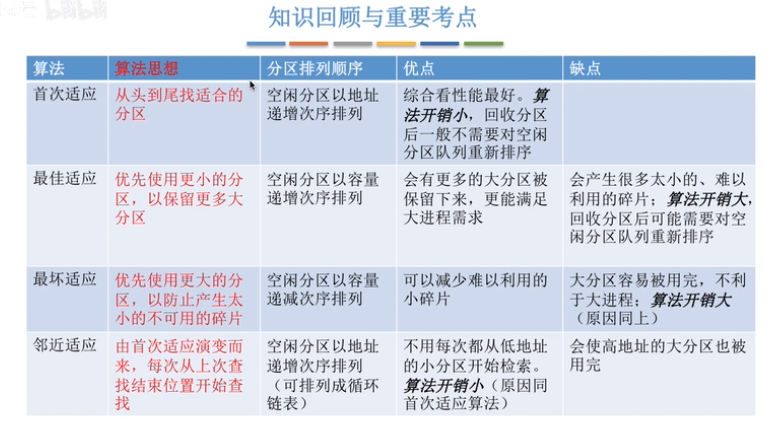展开全文操作系统
• 存储管理——动态分区分配算法的模拟 要求设计主界面以灵活选择某算法，以下算法都要实现： a、首次适应算法 b、循环首次适应算法 c、最佳适应算法 d、最坏适应算法 e、快速适应算法 具体要求： 1）首先由...java UI
• 提出了一种基于分类挖掘的资源动态分配模型和算法,通过资源管理服务器中的守护进程,对集群中的任务动作进行分类挖掘,形成分类规则,用以指导资源的动态分配。实验证明,相比其他分配策略和算法,DRA算法能较好地适应...
• 操作系统——动态分配 写的时间早了，有些许漏洞和不足，请大家不要介意 分配方式可分为四类:单一连续分配、固定分区分配、动态分区分配以及动态可重定位分区分配算法四种方式，其中动态分区分配算法就是此实验的...

操作系统——动态分配

写的时间早了，有些许漏洞和不足，请大家不要介意

分配方式可分为四类:单一连续分配、固定分区分配、动态分区分配以及动态可重定位分区分配算法四种方式，其中动态分区分配算法就是此实验的实验对象。动态分区分配又称为可变分区分配，它是根据进程的实际需要，动态地为之分配内存空间。在实现动态分区分配时，将涉及到分区分配中所用的数据结构、分区分配算法和分区的分配与回收操作这样三方面的问题。动态分区分配又称为可变分区分配，它是根据进程的实际需要，动态地为之分配内存空间。在实现动态分区分配时，将涉及到分区分配中所用的数据结构、分区分配算法和分区的分配与回收操作这样三方面的问题。

大体流程图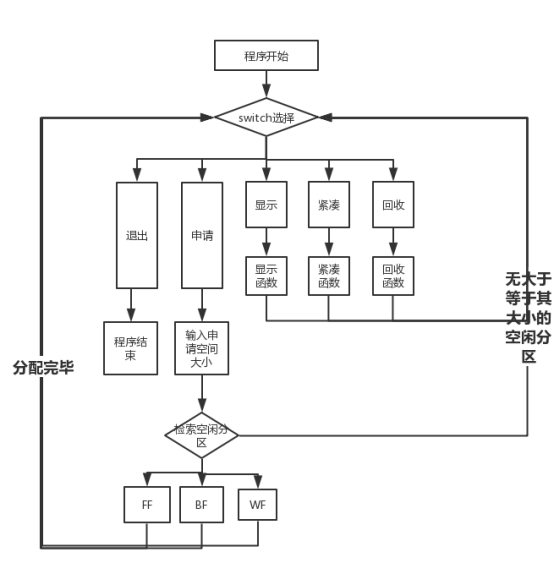算法实现原理

定义的结构体：

struct Partition
{
Partition* Back;//指向上一个分区
int Id;//分区号
int Size;//分区大小
int State;// 分区状态 0空闲 1占用
Partition* Next;//指向下一个分区
};

首次适应算法：

空闲分区以地址递增的次序排列。每次分配内存时顺序查找空闲分区链（或空闲分区表），找到大小能满足要求的第一个空闲分区。

void First_Fit(Partition* Partition_Table_Head, int Size)  //首次适应算法
{
int x = 1;
Partition* p=NULL, * q=NULL;//定义两个指针
while (p)
{
if (p->Size >= Size && p->State == 0) break;

p = p->Next;
}
//利用循环找到一个空闲的块，且其空间大小要大于等于要申请的空间
if (p->Size == Size)  p->State = 1;//若大小恰好合适，只需需改其状态即可

else
{
q = (Partition*)malloc(sizeof(Partition));
(p->Back)->Next = q;
p->Size = p->Size - Size;
q->Back = p->Back;
q->Size = Size;
q->State = 1;
q->Next = p;
p->Back = q;
}
//若大于，则新建一个块，填写其数据，并修改当前块数据，加到其之前
while (p)
{
p->Id = x;
x++;
p = p->Next;
}//x重新给所有分区编号

}

最佳适应算法：

空闲分区按容量递增次序链接。每次分配内存时顺序查找空闲分区链（或空闲分区表），找到大小能满足要求的第一个空闲分区。

void Best_Fit(Partition* Partition_Table_Head, int Size) //最佳适应算法
{
int x = 1, count = 0, Remain_Size = 0;//conut用来保证第一个找到的分区可以直接用，Remain-size用来标识最好的大小
Partition* p = nullptr;
Partition* q = nullptr;//定义两个空指针
while (p)
{

if (p->Size >= Size && p->State == 0)//找到一个空闲区，其大小大于等于要申请的大小
{
if (count == 0) //若是第一个找到的合适分区，则直接赋给q,并计算Remian_size
{
Remain_Size = p->Size - Size;
q = p;
count++;
}
else if (count != 0 && p->Size - Size < Remain_Size)若非第一个找到的合适分区，则比较Remain_size,选择小的重新赋给q
{
Remain_Size = p->Size - Size;
q = p;
}

}

p = p->Next;
}
//同理，利用循环找到“最佳”的那个空闲区
p = q;
if (p->Size == Size)  p->State = 1;

else
{
q = (Partition*)malloc(sizeof(Partition));
(p->Back)->Next = q;
p->Size = p->Size - Size;
q->Back = p->Back;
q->Size = Size;
q->State = 1;
q->Next = p;
p->Back = q;
}

while (p)
{
p->Id = x;
x++;
p = p->Next;
}
//同理，为新的分区前写信息
}

最坏适应算法：

空闲分区按容量递减次序链接。每次分配内存时顺序查找空闲分区链（或空闲分区表），找到大小能满足要求的第一个空闲分区。

void Worst_Fit(Partition* Partition_Table_Head, int Size) //最坏适应算法
{
int x = 1, count = 0, Remain_Size = 0;
Partition* p = nullptr;
Partition* q = nullptr;
while (p)
{

if (p->Size >= Size && p->State == 0)
{
if (count == 0)
{
Remain_Size = p->Size - Size;
q = p;
count++;
}
else if (count != 0 && p->Size - Size > Remain_Size)
{
Remain_Size = p->Size - Size;  //道理同最佳适应算法，只是在这块选择Remain_size大的
q = p;
}

}

p = p->Next;
}

p = q;
if (p->Size == Size)  p->State = 1;

else
{
q = (Partition*)malloc(sizeof(Partition));
(p->Back)->Next = q;
p->Size = p->Size - Size;
q->Back = p->Back;
q->Size = Size;
q->State = 1;
q->Next = p;
p->Back = q;
}

while (p)
{
p->Id = x;
x++;
p = p->Next;
}

}

回收：

对回收一个分区的四种情况，进行处理

{

int x = 1, id;
Partition* p=NULL, * q=NULL;//定义两个空指针
cout << "请输入要回收分区的分区号 ：" << endl;
cin >> id;
while (p)
{
if (p->Id >= id) break;

p = p->Next;
}

p->State = 0;  //根据输入要回收的分区号，使p指向它，并修改其状态为空闲

check_again:	if (p->Back != NULL && (p->Back)->State == 0) //检查上一个分区是否为空，若为空，则合并
{
p->Size = p->Size + (p->Back)->Size;

if ((p->Back)->Back != NULL)	((p->Back)->Back)->Next = p;
else p->Back = NULL;

q = p->Back;
p->Back = (p->Back)->Back;
free(q);
goto check_again;  //再次检查，避免连续的空闲（虽然没可能）
}

if (p->Next != NULL && (p->Next)->State == 0) //检查下一个分区是否为空i，若为空，则合并
{
p->Size = p->Size + (p->Next)->Size;
q = p->Next;

if ((p->Next)->Next != NULL) p->Next = (p->Next)->Next;
else p->Next = NULL;
free(q);
goto check_again; //再次检查，避免连续的空闲（虽然没可能）
}

while (p)
{
p->Id = x;
x++;
p = p->Next;
}  //重新编号

}

算法效果

跳过

首次适应算法、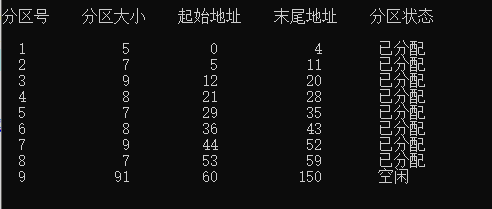回收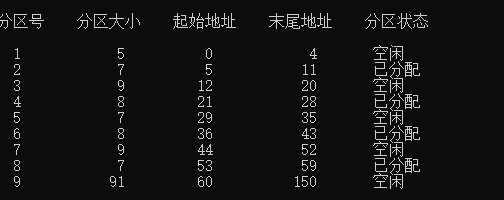回收8最佳适应算法

申请大小为7的分区最坏适应算法

申请大小为5的分区每种算法的优劣

4.1首次适应算法

优点：高址部分的大的空闲分区得到保留，为大作业的内存分配创造了条件；

缺点：（1）每次都是优先利用低址部分的空闲分区，造成低址部分产生大量的外 部碎片

（2）每次都是从低址部分查找，使得查找空闲分区的开销增大；

4.2最佳适应算法

优点：第一次找到的空闲分区是大小最接近待分配内存作业大小的；

缺点:产生大量难以利用的外部碎片

4.3最坏适应算法

优点：效率高，分区查找方便；

缺点：当小作业把大空闲分区分小了，那么，大作业就找不到合适的空闲分区。

展开全文算法 操作系统 c++ 链表
• 设计了一种改进的VM在线动态供应和分配算法（ODAA-VM）。只要用户发现请求或已经被分配的部分VM实例再次可用，便迅速调用ODAA-VM，确定在所要求的时期内为哪些用户分配VM 实例，并保证这些用户在整个被请求期间可以...云计算 在线算法 分配
• 利用银行家算法进行资源分配的报告，包括程序和其他的一套说明都在我这里可以找到匹配的。
• 为了提高WCDMA(wideband code division multiple access)系统中高优先级业务的接入率，提出一种基于优先级的抢占式OVSF(orthogonal variable spreading factor codes)码动态分配算法。该算法中，当新的高优先级业务...
• 操作系统实验的动态分区分配算法java版本的，功能实现。包括分配回收机制，和判断空间是否够，然后再分配，回收利用的情况。动态分区分配算法
• 结合大数据流特征和改进SOM聚类的资源动态分配算法.pdf
• 动态分配算法演示程序（银行家算法） 一、各模块基本算法流程 1、整个银行家算法的思路。先对用户提出的请求进行合法性检查，再进行预分配，利用安全性检查算法进行安全性检查。 2、算法用到的主要数据结构和...

动态分配算法演示程序（银行家算法）

一、各模块基本算法流程

1、整个银行家算法的思路。先对用户提出的请求进行合法性检查，再进行预分配，利用安全性检查算法进行安全性检查。
2、算法用到的主要数据结构和C语言说明。
（1）可利用资源向量avaliable[m][n],m为资源的类型。
（2）最大需求矩阵maxx[m][n],n为进程的数量。
（3）已分配矩阵allocation[m][n]
（4）需求矩阵need[m][n]
（5）申请各类资源数量request[m]
（6）工作向量work[m]
（7）、finish[n]表示系统是否有足够的资源分配给进程，0为否，非0为是
3、银行家算法（主程序）
（1）、系统初始化。输入进程数量，资源种类，各进程已分配、还需求各资源数量，各资源可用数量等。
（2）、输入用户的请求i,即第i个进程请求资源。
（3）、检查用户的请求是否小于还需求的数量，条件是request[j]<need[j]。如果条件不符则提示重新输入，即不允许索取大于需求量.
（4）、检查用户的请求是否小于系统中的可利用资源数量，条件是request[j]<avaliable[j]。如果条件不符则申请失败，阻塞该进程，重新进行进程动态资源申请。
（5）、进行资源的预分配，语句如下:
Allocation[j]+=request[j]
Need[p][j]-=request[j]
Avaliable[j]-=request[j]
3、安全性检查算法（safeCheck()）
（1）、设置两个临时变量。Finish[i]记录进程模拟执行的结束状态，初值为0，如果可以模拟执行结束，则可设为1，也可设为其它非零值以表示执行的先后次序。Work[j]记录模拟执行中资源的回收情况，初值为available[j]的值。
（2）、在进程中查找符合以下条件的进程。条件1：finish[i]=0条件2：need[p][j]<work[j]（3）、如果查找成功则进行资源的模拟回收，语句如下：
for (j = 0;j<m;j++)
work[j] += allocation[i][j];
或查找到的顺序号
（4）、如果查找不成功，则检查所有进程的Ffinish[i]=1，如果有一个为0，则系统不为0，返回不成功

二、流程图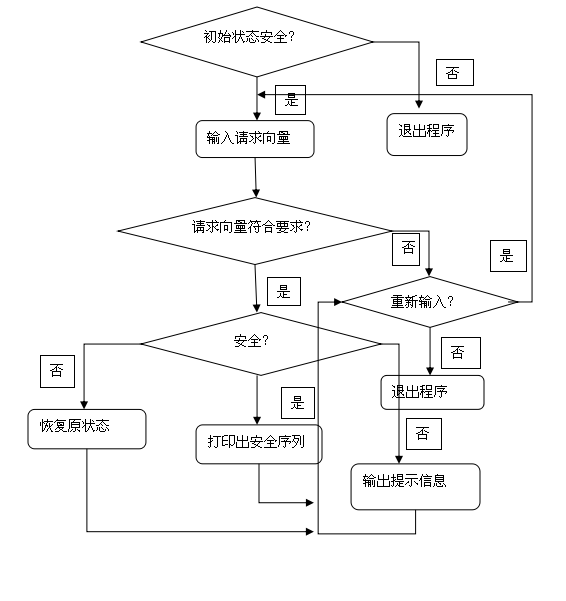三、代码
3、代码
（1）、初始化inint()
#include “stdafx.h”
#include<stdio.h>
#include<stdlib.h>
#include
using namespace std;
int n;//进程的个数
int m, *available, *state, *work, *request, *finish, *safe, *order, *count1;//系统的资源数
int **maxx, **allocation, **need;//最大需求矩阵,分配矩阵，需求矩阵
void inint() //初始化函数
{
int i, j, k;
cout << “请输入此算法涉及到的资源种类:” << ‘\n’;
cin >> m;
cout << “请输入进程的数目:” << ‘\n’;
cin >> n;
available = (int *)malloc(sizeof(int)*m);
work = (int *)malloc(sizeof(int)*m);
state = (int *)malloc(sizeof(int)*n);
finish = (int *)malloc(sizeof(int)*n);
for (i = 0;i<n;i++)
finish[i] = 0;
safe = (int *)malloc(sizeof(int)*n);
for (i = 0;i<n;i++)
safe[i] = 0;
order = (int *)malloc(sizeof(int)*n);
request = (int *)malloc(sizeof(int)*m);
for (i = 0;i<n;i++)
state[i] = 0;
count1 = (int *)malloc(sizeof(int)*n);
cout << “请输入资源的总数目（依次输入）:” << ‘\n’;
for (i = 0;i<m;i++)
cin >> count1[i];
cout << “请输入资源的数目（依次输入）:” << ‘\n’;
for (i = 0;i<m;i++)
cin >> available[i];

maxx = (int**)malloc(sizeof(int*)*n);
for (i = 0;i<n;i++)
*(maxx + i) = (int *)malloc(sizeof(int)*m);
need = (int**)malloc(sizeof(int*)*n);
for (i = 0;i<n;i++)
*(need + i) = (int *)malloc(sizeof(int)*m);
allocation = (int**)malloc(sizeof(int*)*n);
for (i = 0;i<n;i++)
*(allocation + i) = (int *)malloc(sizeof(int)*m);
cout << "请输入最大需求矩阵:" << '\n';
for (i = 0;i<n;i++)
for (j = 0;j<m;j++)
cin >> maxx[i][j];
cout << "请输入需求矩阵:" << '\n';
for (i = 0;i<n;i++)
for (j = 0;j<m;j++)
cin >> need[i][j];
for (i = 0;i<n;i++)
for (j = 0;j<m;j++)
allocation[i][j]=maxx[i][j]-need[i][j];
}

void display() //输出状态函数
{
int i, j;
cout << “当前资源向量：” << ‘\n’;
for (i = 0;i<m;i++)
{
cout << available[i] << ’ ';
}
cout << ‘\n’;
cout<<“";
cout << ‘\n’;
cout << “最大需求矩阵：” << ‘\n’;
for (i = 0;i<n;i++)
{
for (j = 0;j<m;j++)
cout << maxx[i][j] << ’ ';
cout << ‘\n’;
}
cout << ‘\n’;
cout<<"
”;
cout << ‘\n’;
cout << “当前分配矩阵:” << ‘\n’;
for (i = 0;i<n;i++)
{
for (j = 0;j<m;j++)
cout << allocation[i][j] << ’ ';
cout << ‘\n’;
}
cout << ‘\n’;
cout<<“";
cout << ‘\n’;
cout << “当前需求矩阵:” << ‘\n’;
for (i = 0;i<n;i++)
{
for (j = 0;j<m;j++)
cout << need[i][j] << ’ ';
cout << ‘\n’;
}
cout << ‘\n’;
cout<<"
”;
cout << ‘\n’;
}
void roolBack(int p) //回滚函数，恢复到原来状态
{
int j;
for (j = 0;j<m;j++)
{
available[j] += request[j];
allocation[p][j] -= request[j];
need[p][j] += request[j];
}
}
int checkSafe(int k) //安全检查函数，如果安全可形成一个安全序列
{
int i, j;
int flag = 1;
for (i = 0;i<m;i++)
work[i] = available[i];
for (i = 0;i<n;i++)
{
flag = 1;
if (!finish[i])
{
for (j = 0;j<m;j++)
{
if (need[i][j]>work[j])
flag = 0;
}
if (flag)
{
for (j = 0;j<m;j++)
work[j] += allocation[i][j];
finish[i] = 1;
safe[k++] = i;
i = -1;
}
}
}
for (i = 0;i<n;i++)
{
if (!finish[i])
return 0;
}
return 1;
}
int request1(int p) //检查请求向量是否符合要求，小于需求需求向量，//并小于资源向量
{
int flag = 1;
int j;
for (j = 0;j<m;j++)
{
if (request[j]>need[p][j])
{
flag = 0;
}
}
for (j = 0;j<m;j++)
{
if (request[j]>avaliable [p][j])
{
flag = 0;
}
}
return flag;
}
int banker() //银行家算法实现函数
{
int i, j, p;
char ch;
cout << “初始化安全性检查:” << ‘\n’;
int k = 0;
for (i = 0;i<n;i++)
finish[i] = 0;
if (checkSafe(k))
{
cout << “处于安全状态.安全序列为：”;
for (i = 0;i<n;i++)
cout << safe[i] << ’ ';
cout << ‘\n’;
}
else
{
cout << “初始资源出错，退出程序.” << ‘\n’;
return 0;
}
display();
do {
for (i = 0;i<n;i++)
safe[i] = 0;
for (i = 0;i<n;i++)
finish[i] = 0;
cout << “请输入当前请求进程号（例如：p0,输入0）:” << ‘\n’;
cin >> p;
cout << “请输入当前请求向量（例如：请求（1，2，3），输入1，2，3）:” << ‘\n’;
for (i = 0;i<m;i++)
cin >> request[i];
if (request1§)
{
for (j = 0;j<m;j++)
{
available[j] -= request[j];
allocation[p][j] += request[j];
need[p][j] -= request[j];
}
k = 0;
if (checkSafe(k))
{
cout << “处于安全状态.安全序列为：”;
for (i = 0;i<n;i++)
cout << safe[i] << ’ ';
cout << ‘\n’;
}
else
{
roolBack§;
cout << “不安全，请重新输入请求向量.” << ‘\n’;
}
}
else
{
cout << “当前请求向量不符合要求，请重新输入.” << ‘\n’;
}
display();
cout << “是否继续分配?(y or n)”;
cin >> ch;
} while (ch == ‘y’ || ch == ‘Y’);
return 0;
}
int main()
{
cout << “银行家算法” << ‘\n’;
inint();
display();
banker();
return 0;
}

展开全文操作系统 动态分配算法 银行家算法 期末大作业
• 用C/C++实现一个完整的（可变）动态分区管理器，包括分配，回收，分区碎片整理等。希望同学们实现如下功能： 初始化功能：内存状态设置为初始状态。 分配功能：要求至少使用两种算法，用户可以选择使用。 回收...
• 一设计任务 完成存储器动态分区分配算法的模拟实现 二设计思想 在对数据结构有一定掌握程度的情况下设计合理的数据结构来描述存 储空间实现分区存储管理的内存分配功能 应该选择最合适的适应算法 (首 次适应算法最佳...
• 云计算中一种高效的虚拟机在线动态分配算法.pdf
• 云计算中一种高效的虚拟机在线动态分配算法 张丽敏 西安外事学院现代教育技术中心 西安 710077 CA-Provision算法和ODAA-VM的总体结果 电信科学,2015,31(4,106-113.DOI:10.11959/j.issn.1000-0801.2015048
• 针对认知无线电网络的复杂性以及动态性，通过单次博弈很难找到最佳平衡点的问题，提出了一种基于多次博弈的动态频谱分配算法，并通过博弈论的相关原理对该算法中纳什均衡的存在性和唯一性进行了验证。仿真结果表明，...
• 基于FPGA可重构设计中资源动态分配算法研究.pdf
• 《计算机与操作系统（第四版）》动态分区分配算法 1.首次适应算法（first fit） 2.循环首次适应算法（next fit） 3.最佳适应算法（best fit）C语言
• 操作系统的动态分区分配算法（控制台程序），供学习使用。动态分区分配
• 操作系统实验四 目 分区分配算法 实验学时 4学时 目的 通 次 加深 分区分配算法的理解 一步掌握首次适 算法循 首次适 算法 最佳适 算法和最坏适 算法的 方法 内容及要求 描述 程序模 四种 分区分配算法首次适 算法...
• 浙江大学玉泉校区信电楼505室 主要内容 分配问题蛮力法3.4.3 分配问题分支界限法12.2.1 旅行商问题蛮力法3.4.1 旅行商问题分支界限法12.2.3 旅行商问题近似算法12.3.1 背包问题蛮力法3.4.2 背包问题动态
• 移动某些已分配区的内容，使所有进程的分区紧凑在一起，而把空闲分区留在另一端。这种技术称为紧凑（或拼接）。采用紧凑技术的可变分区法称为动态可重定位分区法。该论文为学校学期期末小论文，格式并非标准论文格式...
• 操作系统实验四 动态分区分配算法（内含源代码和详细实验报告），详细介绍：http://blog.csdn.net/xunciy/article/details/79239096操作系统
• 2016 新编操作系统实验四报告 - 动态分区分配算法 操作系统实验报告 实验四 动态分区分配算法 学号: 班级: 姓名: 实验目的 通过这次实验加深对动态分区分配算法的理解进一步掌握首次适应算法 循环首次适应算法最佳...
• 课题八:存储管理---动态分区分配算法的模拟： 要求设计主界面以灵活选择某算法，且以下算法都要实现：首次适应算法、循环首次适应算法、最佳适应算法；...